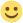Repaint Tutorial (now with Colour-Code Wireframe) - How To Repaint Fernbus

Welcome to the new TML-Studios Community! Please read through the Community Rules and Guidelines and Tips & Tricks. Have fun!
• Hi there,

I thought a tutorial showing you how to make your own repaint may be of use, so here it is.

I hope it helps+=+=+=+=+=+=+=+=+=+=+=+=+=+=+=+=+=+=+=+=+=+=+=+=+=+=+=+=+=+=+=+=+=+=+=+=+=+=+=+=+=+=+=+=+=+=+=+=+=+=+=+=+=+=+=+=+=+=+=+=+=+=+=

UPDATE

I hope it helpsIn an attempt to make repaints easier I have created a colour-coded wireframe see here:

It's not 100% finished yet but if enough people want it and hit the like button I will finish it properly, do the other coach and upload both here.

If it's not useful then OK no worriesEdited 3 times, last by Sim UK ().